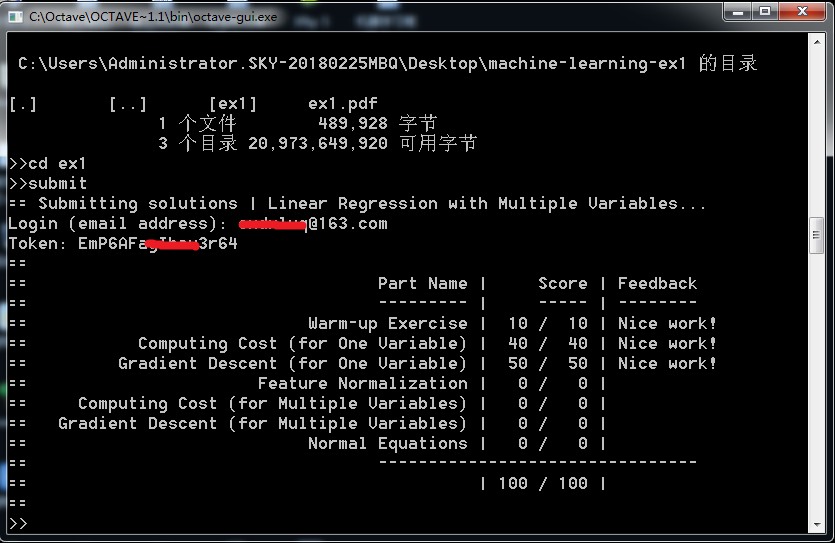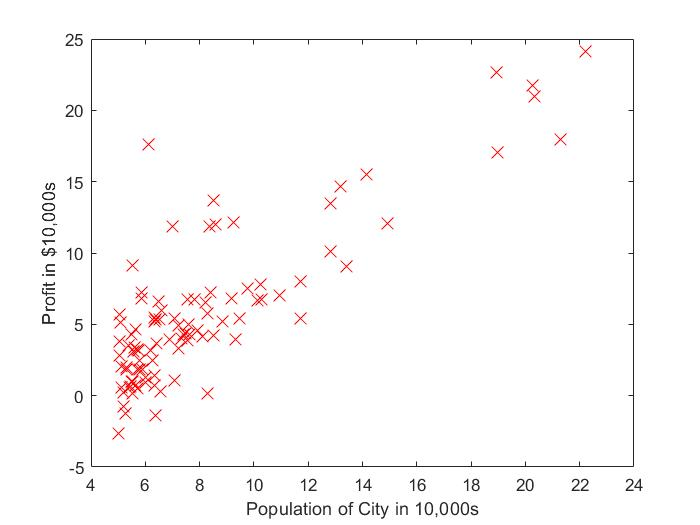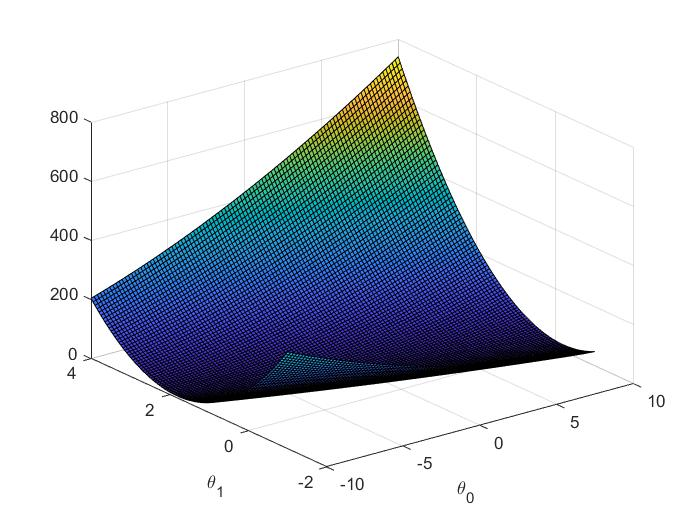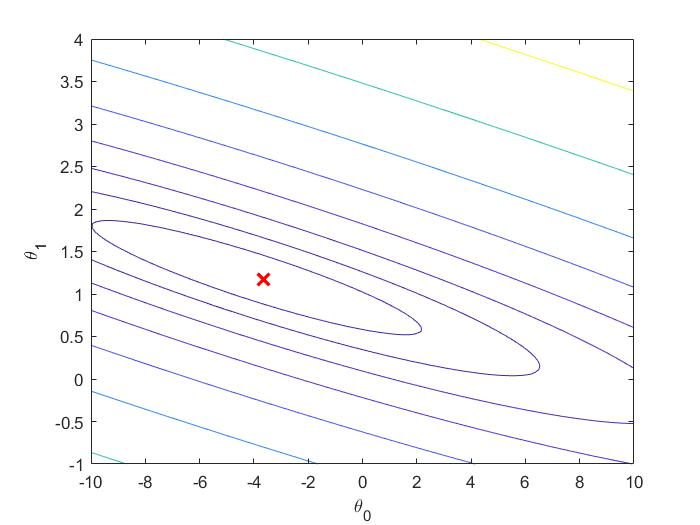• 吴恩达机器学习课程的python语言代码，包括大佬改进的代码，jupyter notebook完美运行
•机器学习
• 吴恩达机器学习coursera题目+答案，matlab版。感觉还可以，可以跟课程一起学习。机器学习
• Course mechine-learning 斯坦福 吴恩达 机器学习 编程作业答案
• 机器学习课后作业文档说明和答案解析，配套2014年的视频
• 斯坦福教授、人工智能和机器学习领域国际最权威学者之一吴恩达机器学习课程的全部编程作业，内不含答案。
• 吴恩达教授machine learning 的exercise以及代码与解析
• 题目+答案，很好用。解决了在线看coursera课程作业下载不了的问题。
• 吴恩达网易云机器学习公开课：https://study.163.com/course/courseMain.htm?courseId=1004570029 课程课后编程答案，使用Matlab编程。吴老师的课程浅显易懂，代码框架已书写完成，主要需要学生自行填写必要的函数体...
• 包含吴恩达机器学习神经网络练习及答案，使用octave编写与课程一致，答案已经根据要求填充到相应的函数文件中。
• 吴恩达斯坦福机器学习课程作业（matlab完整版）,吴恩达斯坦福机器学习课程作业（matlab完整版）,亲测亲测
• 本资源是吴恩达老师讲授的机器学习网络公开课练习，共8个练习，包括原始练习和作者整理（带答案）的代码。
• 该资源是吴恩达公开课后的8次作业的习题+编程答案，编程答案分为python版本和matlab版本
• 本资源中，包含吴恩达机器学习线性回归练习及答案，使用octave编写与课程一致，答案已经根据要求填充到相应的函数文件中。
• 机器学习所有课后作业 大大的 只看没用 做了练习才有点儿用 课后答案可以网上找 练习题目很有必要
•吴恩达 机器学习
• 本资源是coursera课程中斯坦福吴恩达教授的机器学习课程中课后编程习题的资源及答案，全部代码是本人积累验证得来，均为满分答案，可供各位学者参考。
• 在我提交吴恩达的ML作业的时候，出现了以下错误代码： [error] submission with curl() was not successful !! Submission failed: 错误使用 submitWithConfiguration>validateResponse (line 158) Grader ...

在我提交吴恩达的ML作业的时候，出现了以下错误代码：

[error] submission with curl() was not successful

!! Submission failed: 错误使用 submitWithConfiguration>validateResponse (line 158)

Function: validateResponse
LineNumber: 158

我的环境是Matlab R2014a，我按照网上的修改方法解决了很多次，无法解决。

最后用Octave提交,竟然成功了。。。（我的Octave版本是Octave4.2.1），下载链接：点击这里下载，进入后选择相应的版本，我的是64位的。============= Note ================

Note: 每个人的错误可能都不一样，多在网上找找相应的解决办法即可。

如果你通过此方法解决了问题，欢迎点个赞啊！笔芯！

展开全文机器学习 吴恩达
• Coursera吴恩达机器学习课程第四周编程作业，含有详细解析，有任何不明白的问题下载后可以咨询博主机器学习
• 已经学习吴恩达机器学习课程，这个是编程作业，带答案自己写的，所有答案全部通过。吴恩达
• 吴恩达coursera机器学习课程课后习题，可以成功提交coursera审核
• 吴恩达机器学习作业答案（python版）

千次阅读 多人点赞 2020-02-27 17:08:14
上学期学了吴恩达机器学习课程，用python完成了各章的作业。在这里为了帮助初学者少走一些弯路，把我的作业代码分享给大家。 首先感谢Cowry5作者，我刚学的时候主要参考他的代码，大家也可以去他的主页找来各个...

上学期学了吴恩达的机器学习课程，用python完成了各章的作业。在这里为了帮助初学者少走一些弯路，把我的作业代码分享给大家。

首先感谢Cowry5作者，我刚学的时候主要参考他的代码，大家也可以去他的主页找来各个章节的答案来参考 https://blog.csdn.net/Cowry5

可以说我的代码与Cowry5的代码相似度有90%，毕竟是参考他的代码来写的。区别是我在代码中添加了许多注释（网上大部分代码都是没有注释的，这可能会使新手读起来晦涩难懂），可以帮助大家来理解。其中Cowry5作者的代码中在神经网络章节有一个小错误，我的代码中已经更改。

我把各个章节的答案代码以及需要用到的数据文件打包传到了百度网盘中，大家需要的话可以来下载。

链接：https://pan.baidu.com/s/1D6YgmdTzxp4AtXQ67lu7VA
提取码：kmpf

下载后把数据文件放到系统的环境变量中，就可以用了。.py结尾的文件即题目答案，用代码编辑器打开即可。

最后祝大家高效地完成机器学习课程的学习。

展开全文• 本文件是网易云课堂吴恩达老师机器学习课程课后作业的Python实现，本部分是线性回归的作业，其他部分的作用也会陆续上传。
• 斯坦福大学吴恩达教授的课件上的同步练习，每个章节都有对应的习题。机器学习
• 吴恩达机器学习作业1-linear regression。matlab代码。
• 作业ex1题目下载地址ex1 一. 问题背景：假如你是餐馆老板，已知若干城市中人口和利润的数据（ex1data1.txt），用线性回归方法计算该去哪个城市发展。 ex1.m是官方提供的入口函数，通过该函数补全其他函数的代码。 ...

作业ex1题目及答案源码下载地址ex1题目及答案

一. 单变量线性回归

问题背景：假如你是餐馆老板，已知若干城市中人口和利润的数据（ex1data1.txt），用线性回归方法计算该去哪个城市发展。 ex1.m是官方提供的入口函数，通过该函数补全其他函数的代码。

1. warmUpExercise.m：输出5*5的单位矩阵
A = eye(5);

Matlab中，eye(n) 返回n*n的单位矩阵，eye(m,n) 返回m*n的单位矩阵

1. plotData.m：给定x、y两列向量，画出相应点
figure; % open a new figure window
plot(x, y, 'rx', 'MarkerSize', 10); % Plot the data
ylabel('Profit in \$10,000s'); % Set the y   axis label
xlabel('Population of City in 10,000s'); % Set the x   axis label1. computeCost.m：计算代价
假设hypothesis函数为
h θ = θ T x = θ 0 + θ 1 x 1 h_\theta=\theta^Tx=\theta_0+\theta_1 x_1
一共m个数据，代价函数为
J ( θ ) = 1 2 m ∑ i = 1 m ( h θ ( x ( i ) ) − y ( i ) ) 2 J(\theta)=\frac{1}{2m}\sum_{i=1}^m(h_\theta(x^{(i)})-y^{(i)})^2
J = sum((X * theta - y).^2) / (2*m);

为方便求导，将 J ( θ ) J(\theta) 写成如下形式
J ( θ ) = 1 2 m [ ( h θ ( x ( 1 ) ) − y ( 1 ) ) 2 + ( h θ ( x ( 2 ) ) − y ( 2 ) ) 2 + . . . ] J(\theta)=\frac{1}{2m}[(h_\theta(x^{(1)})-y^{(1)})^2+(h_\theta(x^{(2)})-y^{(2)})^2+...]
θ 1 \theta_1 求偏导得
∂ ∂ θ 1 J ( θ ) = 1 2 m [ 2 ( h θ ( x ( 1 ) ) − y ( 1 ) ) x 1 ( 1 ) + 2 ( h θ ( x ( 2 ) ) − y ( 2 ) ) x 1 ( 2 ) + . . . ] = 1 m ∑ i = 1 m ( h θ ( x ( i ) ) − y ( i ) ) x 1 ( i ) \begin{aligned} \frac{\partial}{\partial{\theta_1} }J(\theta)=&amp;\frac{1}{2m}[2(h_\theta(x^{(1)})-y^{(1)})x^{(1)}_1+2(h_\theta(x^{(2)})-y^{(2)})x^{(2)}_1+...]\\ =&amp;\frac{1}{m}\sum_{i=1}^m(h_\theta(x^{(i)})-y^{(i)})x^{(i)}_1 \end{aligned}
所以有
θ j : = θ j − α 1 m ∑ i = 1 m ( h θ ( x ( i ) ) − y ( i ) ) x j ( i ) , ( j = 0 , 1 ) \theta_j:=\theta_j-\alpha\frac{1}{m}\sum_{i=1}^m(h_\theta(x^{(i)})-y^{(i)})x^{(i)}_j,(j=0,1)
theta(1) = theta(1) - alpha / m * sum(X * theta_s - y);
theta(2) = theta(2) - alpha / m * sum((X * theta_s - y) .* X(:,2));
theta_s=theta;

迭代多次后便可得到理想的 θ \theta 值。接下来的根据x预测y值，以及绘制三维图、等高线图题目中已给出，这里不再赘述。matlab中的画图函数surf和画等高线图函数contour可以自行百度。二、多变量线性回归

问题背景：卖房子，已知一系列数据，根据房屋大小和卧室数量两个特征，预测房子价钱，数据在（ex1data2.txt）。

1. featureNormalize.m：数据特征归一化，因为房子大小和卧室数量不在一个数量级，直接计算收敛速度慢，所以先采用0均值标准化，归一化公式如下，详细介绍见线性回归与特征归一化(feature scaling)
z = x − μ σ z=\frac{x-\mu}{\sigma}
均值方差根据matlab容易求得，代码如下
mu = mean(X);       %  mean value
sigma = std(X);     %  standard deviation
X_norm  = (X - repmat(mu,size(X,1),1)) ./  repmat(sigma,size(X,1),1);

1. computeCostMulti.m：计算代价
假设hypothesis函数为
h θ = θ T x = θ 0 + θ 1 x 1 + θ 2 x 2 h_\theta=\theta^Tx=\theta_0+\theta_1 x_1+\theta_2 x_2
一共m个数据，代价函数为
J ( θ ) = 1 2 m ∑ i = 1 m ( h θ ( x ( i ) ) − y ( i ) ) 2 J(\theta)=\frac{1}{2m}\sum_{i=1}^m(h_\theta(x^{(i)})-y^{(i)})^2
J = sum((X * theta - y).^2) / (2*m);

J ( θ ) J(\theta) θ j \theta_j 求偏导得
∂ ∂ θ j J ( θ ) = 1 2 m [ 2 ( h θ ( x ( 1 ) ) − y ( 1 ) ) x j ( 1 ) + 2 ( h θ ( x ( 2 ) ) − y ( 2 ) ) x j ( 2 ) + . . . ] = 1 m ∑ i = 1 m ( h θ ( x ( i ) ) − y ( i ) ) x j ( i ) \begin{aligned} \frac{\partial}{\partial{\theta_j} }J(\theta)=&amp;\frac{1}{2m}[2(h_\theta(x^{(1)})-y^{(1)})x^{(1)}_j+2(h_\theta(x^{(2)})-y^{(2)})x^{(2)}_j+...]\\ =&amp;\frac{1}{m}\sum_{i=1}^m(h_\theta(x^{(i)})-y^{(i)})x^{(i)}_j \end{aligned}
所以有
θ j : = θ j − α 1 m ∑ i = 1 m ( h θ ( x ( i ) ) − y ( i ) ) x j ( i ) , ( j = 0 , 1 ) \theta_j:=\theta_j-\alpha\frac{1}{m}\sum_{i=1}^m(h_\theta(x^{(i)})-y^{(i)})x^{(i)}_j,(j=0,1)
θ = [ θ 0 , θ 1 , θ 2 ] T \theta=[\theta_0,\theta_1,\theta_2]^T X ∈ R m × 3 , Y ∈ R m X\in R^{m\times3},Y\in R^{m}
θ = θ − α 1 m X T ( X θ − Y ) \theta=\theta-\alpha\frac{1}{m} X^T(X\theta-Y)
theta = theta - alpha / m * X' * (X * theta - y);

1. 根据迭代n次后得到的 θ \theta ，计算1650平方英尺、3个卧室的房子价钱
price = [1 (([1650 3]-mu) ./ sigma)] * theta ;

三、用正规方程（解析解）直接计算 θ \theta

根据 ∇ θ J ( θ ) = 0 \nabla_\theta J(\theta)=0 求得解析解如下（具体过程见视频课程）
θ = ( X T X ) − 1 X T Y \theta=(X^T X)^{-1}X^T Y
代码为

theta = pinv( X' * X ) * X' * y;

不再需要使用归一化处理数据。

解析解和梯度下降都可以很好的进行预测，上述实验数据虽然最后得到的 θ \theta 不同，但是预测结果一致。解析方法在数据量极大时存在时间效率问题。具体可见正规方程normal equation

展开全文机器学习 吴恩达课程
• 吴恩达机器学习笔记（一） 监督学习 非监督学习 代价函数cost function 梯度下降和线性回归gradient descent and linear regression 梯度下降 多变量梯度下降 特征标准化变量归一法feature normalization 关于alpha...

吴恩达机器学习笔记（一）

标签（空格分隔）： 机器学习

一.机器学习简介

1.机器学习的定义

对于一个程序，给它一个任务T和一个性能测量方法P，如果在经验E的影响下，P对T的测量结果得到了改进，那么就说该程序从E中学习

分类内容例子
监督学习回归 在已有数据中寻找规律，解决类似问题。预测房价
无监督学习聚类，独立组件分析寻找混合数据之间的区别进行分类。区分声音
强化学习回报函数，自我学习优化算法。机器人运动。

作业软件：Matlab/Octave

2.监督学习

在监督式学习中，我们给了一个数据集，并且已经知道我们的正确输出应该是什么样子，并且有输入和输出之间有关系的想法。

监督学习问题分为“回归(classification)”和“分类(regression)”问题。

• 在回归问题中，我们试图预测连续输出中的结果，这意味着我们试图将输入变量映射到某个连续函数。
• 在分类问题中，我们试图预测离散输出的结果。意味着我们将输入变量映射到离散类别。

3.非监督学习

• 无监督的学习使我们能够很少或根本不知道我们的结果应该是什么样子。
• 我们可以从数据中推导出结构，我们不一定知道变量的影响。
• 我们可以通过基于数据中变量之间的关系对数据进行聚类来推导出这种结构。
• 在无监督学习的基础上，没有基于预测结果的反馈。

例：

• 聚类：搜集一百万个不同的基因，并找到一种方法，将这些基因自动分组，这些基因组通过不同的变量（例如寿命，位置，角色等）相似或相关。

• 非聚类：“鸡尾酒会算法”，可以让你在混乱的环境中找到结构。 （即在鸡尾酒会上从声音网格中识别个别的声音和音乐）。

回归与分类的区分
* 当我们试图预测的目标变量是连续的，比如在我们的住房例子中，我们把学习问题称为回归问题。
* 当y只能接受少量的离散值时（比如，如果考虑到居住面积，我们想要预测一个住宅是房子还是公寓），我们称之为分类问题

代价函数cost function

J(θ0,θ1)=12mi=1m(yi~yi)2=12mi=1m(hθ(xi)yi)2

这个函数被称为“平方误差函数”或“均方误差”。
平方项求导有常数2，增加1_2系数，以方便计算梯度下降。

示例：
假设(hypothesis):

hθ(x)=θ0+θ1x

参数(parameter):
θ0,θ1

代价函数(cost function):
J(θ0,θ1)=12mi=1m(yi~yi)2=12mi=1m(hθ(xi)yi)2

对于多变量还可以写做：

J(θ)=12m(Xθy⃗ )T(Xθy⃗ )()

目标(goal):

minimizeJ(θ0,θ1)

1.梯度下降

重复运算直至收敛：(alpha为学习速率(learning rate))

θj:=θjαααθjJ(θ0,θ1)

同时对两个theta赋值：
temp0=θ0αααθ0J(θ0,θ1) $temp_0=\theta_0-\alpha\frac{\alpha} {\alpha\theta_0}J(\theta_0,\theta_1)$
temp1=θ1αααθ1J(θ0,θ1) $temp_1=\theta_1-\alpha\frac{\alpha} {\alpha\theta_1}J(\theta_0,\theta_1)$
然后将temp赋值给theta循环运行直至算法收敛

• 向量(vector)：一个n*1的矩阵(matrix)
• 只有同维度的矩阵才能相加，对应的数字相加
• 实数与整数相乘除直接把矩阵的每一位乘除即可
• 矩阵相乘必须前一个的列数(columns)等于后一个的行数(row)
acebdf+[xy]=ax+bycx+dyex+fy

逆矩阵：m*m的矩阵与其逆矩阵相乘为单位矩阵 (identity matrix)

AA1=I

转置4 877777777777777矩阵：
A=acebdfAT=[abcdef]

输入的变量：

• n:特征(feature)数量
• m: 训练集数量
• x(i)= $x^{(i)}=$第i个训练集
• x(i)j= $x^{(i)}_j=$第i个训练集第j个特征的参数
• hθ(x)=[θ0θ1θn]x0x1xn=θ $h_\theta(x)=\left[ \begin{array}{ccc} \theta_0 & \theta_1&\cdots&\theta_n\\ \end{array} \right]\left[ \begin{array}{c} x_0 \\ x_1\\\cdots\\x_n \end{array} \right]=\theta$

2.多变量梯度下降：

重复运行直至收敛：｛

θj:=θjα1mi=1m(hθ(x(i))y(i))x(i)j(for j:=0...n)

变量形式可以写作：

θ=θαmXT(Xθy⃗ )()

3.特征标准化、变量归一法（feature normalization）：

防止变量数值差距过大难以收敛，将数据可以缩放到一个合适的范围

xi=xiuisi

ui $u_i$是数据的平均值, si $s_i$是数值的范围（最大值-最小值）,或者是标准差
例如:如果数据范围为100~2000，平均值为1000
则： xi=xi10001900 $x_i=\frac{x_i-1000}{1900}$

mean(X(:,i))与std(X(:,i))可以分别计算第i列的X矩阵的平均数与平方差

4.关于 α $\alpha$学习速率：

如果 α $\alpha$过大，那么 J(θ) $J(\theta)$可能越来越大甚至不收敛
如果 α $\alpha$过小，那么 J(θ) $J(\theta)$迭代速率会非常慢
一般认定如果 J(θ) $J(\theta)$迭代中下降小于一个值，例如1e-3，则判定函数收敛，但是实际应用中很难确定一个合适的判定范围

5.正规方程(normal equations)

类似与一元二次方程对方程直接求导找到最小值

θ=(XTX)1XTy()

• X为m*(n+1)的矩阵
• y是表达结果的一个m*1的矩阵
如果使用正则方程则不需要进行变量归一化
梯度下降法正则方程法
需要选择 α $\alpha$不需要选择 α $\alpha$
需要多次迭代不需要多次迭代
当特征值很多时很有效(约为 O(Kn2) $O(Kn^2)$当特征特别多时运算非常慢(约为 O(n3) $O(n^3)$)

* 当n< 105 $10^5$使用正则方程
* 当n> 108 $10^8$使用梯度下降

如果发现矩阵X不可逆，则查看是否矩阵中有冗余特性，删除其一即可
如果特征太多，则删除一些特征或者使用正则化

三.实际使用中重要的几个式子：

J(θ)=12m(Xθy⃗ )T(Xθy⃗ )(cost function)

θ=(XTX)1XTy(normal equations)

xi=xi10001900(feature normalization)

mean(X(:,i))与std(X(:,i))可以分别计算第i列的X矩阵的平均数与平方差

附上作业答案下载地址（包含附加题）

展开全文机器学习 吴恩达
• 吴恩达机器学习课后习题ex1

千次阅读 2019-07-06 23:43:53
参考学习文章： https://blog.csdn.net/Mrs_Ivory/article/details/91449797 数据ex1data.txt: 6.1101,17.592 5.5277,9.1302 8.5186,13.662 7.0032,11.854 5.8598,6.8233 8.3829,11.886 7.4764,4.3483 8.5781,...吴恩达机器学习...